Immediately get Chapter Wise Tamilnadu State Board Solutions for 11th Economics to gain more marks and start Quick Revision of all Chapters. All Chapters Pdf is provided along with the Questions and Answers. You Can Dowload Samacheer Kalvi 11th Economics Book Solutions Questions and Answers for Chapter wise are given with a clear explanation. Tamilnadu State Board Solutions for 11th Economics Chapter 6 Distribution Analysis Questions and Answers is for free of cost.

## Tamilnadu Samacheer Kalvi 11th Economics Solutions Chapter 6 Distribution Analysis

Tamilnadu State Board Solutions for 11th Economics Chapter 6 Distribution Analysis Questions and Answers PDF has all given in Chapter Wise Section. Check Out daily basis with Tamilnadu State Board Solutions 11th Economics PDF will help to improve your score. Improve your level of accuracy to answer a question by reading with Samacheer Kalvi 11th Economics Book Solutions Questions and Answers PDF.

### Samacheer Kalvi 11th Economics Distribution Analysis Text Book Back Questions and Answers

Part – A
Multiple Choice Questions

Question 1.
In Economics, distribution of income is among the
(a) factors of production
(b) individual
(c) firms
(d) traders
Answer:
(a) factors of production

Question 2.
Theory of distribution is popularly known as ………………………..
(a) Theory of product – pricing
(b) Theory of factor – pricing
(c) Theory of wages
(d) Theory of Interest
Answer:
(b) Theory of factor-pricing

Question 3.
Rent is the reward for the use of
(a) capital
(b) labour
(c) land
(d) organization
Answer:
(c) land

Question 4.
The concept of ‘Quasi – Rent’ is associated with ………………………
(a) Ricardo
(b) Keynes
(c) Walker
(d) Marshall
Answer:
(d) Marshall

Question 5.
The Classical Theory of Rent was propounded by
(a) Ricardo
(b) Keynes
(c) Marshall
(d) Walker
Answer:
(a) Ricardo

Question 6.
‘Original and indestructible powers of the soil’ is the term used by ……………………….
(a) J.S.Mill
(b) Walker
(c) Clark
(d) Ricardo
Answer:
(d) Ricardo

Question 7.
The reward for labour is
(a) rent
(b) wage
(c) profit
(d) interest
Answer:
(b) wage

Question 8.
Money wages are also known as ……………………….
(a) Real wages
(b) Nominal wages
(c) Original wages
(d) Transfer wages
Answer:
(b) Nominal wages

Question 9.
Residual Claimant Theory is propounded by
(a) Keynes
(b) Walker
(c) Hawley
(d) Knight
Answer:
(b) Walker

Question 10.
The reward is given for the use of capital ………………………
(a) Rent
(b) Wage
(c) Interest
(d) Profit
Answer:
(c) Interest

Question 11.
Keynesian Theory of interest is popularly known as
(a) Abstinence Theory
(b) Liquidity Preference Theory
(c) Loanable Funds Theory
(d) Agio Theory
Answer:
(b) Liquidity Preference Theory

Question 12.
According to the Loanable Funds Theory, supply of loanable funds is equal to …………………………
(a) S + BC + DH + DI
(b) I + DS + DH + BM
(c) S+ DS + BM+ DI
(d) S + BM + DH + DS
Answer:
(a) S + BC + DH + DI

Question 13.
The concept of meeting unexpected expenditure according to Keynes is
(a) Transaction motive
(b) Precautionary motive
(c) Speculative motive
(d) Personal motive
Answer:
(b) Precautionary motive

Question 14.
The distribution of income or wealth of a country among the individuals are ………………………….
(a) Functional distribution
(b) Personal distribution
(c) Goods distribution
(d) Services distribution
Answer:
(b) Personal distribution

Question 15.
Profit is the reward for
(a) land
(b) organization
(c) capital
(d) labour
Answer:
(b) organization

Question 16.
Innovation Theory of profit was given by …………………………..
(a) Hawley
(b) Schumpeter
(c) Keynes
(d) Knight
Answer:
(b) Schumpeter

Question 17.
Quasi-rent arises in
(a) Man-made appliances
(b) Homemade items
(c) Imported items
(d) None of these
Answer:
(a) Man-made appliances

Question 18.
“Wages as a sum of money are paid under contract by an employer to a worker for service rendered”- Who said this?
(a) Benham
(b) Marshall
(c) Walker
(d) J.S.Mill
Answer:
(a) Benham

Question 19.
Abstinence Theory of Interest was propounded by
(a) Alfred Marshall
(b) N.W Senior
(c) Bohm-Bawerk
(d) Knut Wicksell
Answer:
(b) N.W Senior

Question 20.
Loanable Funds Theory of Interest is called as …………………………
(a) Classical Theory
(b) Modem Theory
(c) Traditional Theory
(d) Neo-Classical Theory
Answer:
(d) Neo-Classical Theory

Part – B
Answer the following questions in one or two sentences

Question 21.
What is meant by distribution?
Answer:
Distribution means division of income among the four factors of production as rent, wage, interest and profit.

Question 22.
Mention the types of distribution?
Answer:
Personal Distribution: Personal Distribution is the distribution of national income among the individuals.
Functional Distribution: Functional Distribution means the distribution of income among the four factors of production namely land, labour, capital and organization for their services in production process.

Question 23.
Define ‘Rent’.
Answer:
Rent is that part of payment made by a tenant to his landlords for the use of land only.

Question 24.
Distinguish between real and money wages.
Answer:
Real wages :
Wages paid in terms of goods and services. It refers to the purchasing power of money wages.

Money wages :
Nominal wages are referred to as wages paid in terms of money.

Question 25.
What do you mean by interest?
Answer:

1. Interest is the reward paid by the borrower to the lender for the use of capital.
2. Interest is the price paid for the use of capital in any market.
3. Generally speaking, interest is a payment made by a borrower to the lender for the money borrowed.

Question 26.
What is profit?
Answer:
Profit is the amount left with the entrepreneur after he has made payments for all the other factors of production.

Question 27.
State the meaning of liquidity preference?
Answer:

1. Liquidity preference means the preference of the people to hold wealth in the form of liquid cash rather than in other non – liquid assets like bonds, securities, bills of exchange, land, building, gold etc.
2. “ Liquidity Preference is the preference to have an amount of cash rather than of claims against others”.

Part – C
Answer the following questions in One Paragraph

Question 28.
What are the motives of demand for money?
Answer:
1. Transaction motive :
It relates to the desire of the people to hold cash for the current transactions.
Mt=f (y)

2. Precautionary motive :
It relates to the desire of the people to hold cash to meet unexpected or unforeseen expenditures.
Mp = f (y)

3. Speculative motive :
It relates to the desire of the people to hold cash in order to take advantage of market movements regarding future changes in the price of bonds and securities in the capital market.
Ms = f (i)

Question 29.
List out the kinds of wages:
Answer:
Wages are divided into four types.

1. Nominal Wages or Money Wages: Nominal wages are referred to the wages paid in terms of money.
2. Real Wages: Real wages are the wages paid in terms of goods and services. Hence, real wages are the purchasing power of money wages.
3. Piece Wages: Wages that are paid on the basis of quantum of work done.
4. Time Wages: Wages that are paid on the basis of the amount of time that the worker works.

Question 30.
Distinguish between rent and quasi-rent
Answer:
Rent:

1. Rent accrues to land
2. The supply of land is fixed forever
3. It enters into price

Quasi – rent:

1. Quasi-rent accrues to man-made appliances
2. The supply of man made appliances is fixed for a short period only
3. It does not enter into price.

Question 31.
Briefly explain the Subsistence Theory of Wages?
Answer:
The subsistence theory of wages:

1. Subsistence theory of wages is one of the oldest theories of wages.
2. According to this theory, wage must be equal to the subsistence level of the labourer and his family.
3. Subsistence means the minimum amount of food, clothing and shelter which workers and their family require for existence.
4. If workers are paid higher wages than the subsistence level, the workers would be better off and they will have large families.
5. Hence, the population would increase.
6. When the population increases, the supply of labourer would increase and therefore, wages will come down.
7. If wages are lower than the subsistence level, there would be a reduction in population and thereby the supply of labour falls and wages increase to the subsistence level.

Question 32.
State the Dynamic Theory of Profit.
Answer:

• J.B. Clark propounded this theory in 1900. To him, profit is the difference between price and cost of production of the commodity.
• Profit is the reward for dynamic changes in society. He points out that, profit cannot arise in a static society. In static society everything remains stationary.
• The following changes take place in a dynamic society.
1. Population is increasing.
2. Volume of capital is increasing.
3. Methods of production are improving.
4. Forms of industrial organization are changing.
5. The wants of consumer are multiplying.

Question 33.
Describe briefly the Innovation Theory of Profit?
Answer:
Innovation Theory of Profit:

1. The innovation theory of profit was propounded by Joesph. A.Schumpeter.
2. Schumpeter says an entrepreneur is not only an undertaker of a business but also an innovator in the process of production.
3. Profit is the reward for “innovation”.
4. According to Schumpeter, an innovation may consist of the following:
• Introduction of a new product.
• Introduction of a new method of production.
• Opening up of a new market.
• Discovery of new raw materials
• Reorganization of an industry / firm.

When any one of these innovations is introduced by an entrepreneur, it leads to reduction in the cost of production and thereby brings profit to an entrepreneur. To obtain profit continuously, the innovator needs to innovate continuously. The real innovators do so. Imitative entrepreneurs cannot innovate.

Question 34.
Write a note on the Risk-bearing Theory of Profit.
Answer:

• Risk – the bearing theory was propounded by F.B.Hawley in 1907.
• Profit is the reward for “risk-taking” in business.
• Every business involves some risks. So risk-taking is an essential function of the entrepreneur and is the basis of profit.
• Higher the risks, the greater are the profit.
• Profit induces entrepreneurs to undertake risks.

Part – D
Answer the following questions in about a page

Question 35.
Explain the Marginal Productivity Theory of Distribution.
Answer:
Marginal productivity theory of distribution was developed by Clark, Wickseed and Walras. This theory explains how the prices of various factors of production are determined. This theory is also known as “General theory of distribution” or “National dividend theory of distribution”.
Assumptions:

1. All the factors of production are homogenous and can be substituted for each other.
2. There is perfect competition in both factor and product market.
3. There is perfect mobility and full employment of factors of production.
4. This theory is applicable only in the long-run.
5. The entrepreneurs aim at profit maximization.
6. There is no government intervention and no technological change.

Explanation of the theory :
Each factor is rewarded according to its marginal productivity.

Marginal product:
The marginal product of a factor of production means the addition made to the total product by employment of an additional unit of that factor.

Marginal physical product (MPP) :
The MPP of a factor is the increment in the total product obtained by the employment of an additional unit of that factor.

Value of marginal product (VMP):
VMP = MPP x price

Statement of the theory:

1. The price of a factor of production depends upon its productivity.
2. The price of a factor is determined by and will be equal to marginal revenue product of that factor.
3. Under certain conditions, the price of a factor will be equal to both average and marginal products of that factor.

Marginal productivity under perfect competition: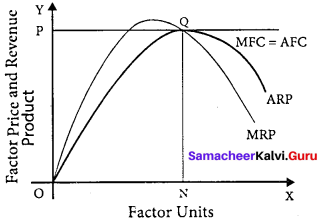X- axis represents factor units and Y-axis represents the factor price and revenue product.
MRP – Marginal Revenue Product Curve
ARP – Average Revenue Product Curve
AFC – Average Factor cost Curve
MFC – Marginal Factor cost Curve
AFC – is horizontal under perfect competition and MFC coincides with it.
When there is perfect competition in the factor market, the firm is in equilibrium only when MFC = MRP
At the point Q by employing ON units of factors and paying OP price (NQ) where MFC = MRP
At Q, MRP = ARP
Price of the factor (NQ) = Marginal revenue
Product (NQ) = Average revenue product (NQ)
There is no exploitation of factors under perfect competition.
Beyond the point Q the price paid to the factor is more than marginal revenue product and average revenue product, so employer do not employ the factors.

Question 36.
Illustrate the Ricardian Theory of Rent.
Answer:
The classical theory of rent is called “Ricardian theory of rent”.
Definition :
Rent is that portion of the produce of the earth which is paid to the landlord for the use of the original and indestructible powers of the soil.

Assumptions :

1. Land differs in fertility.
2. The law of diminishing returns operates in agriculture.
3. Rent depends upon fertility and location of land.
4. Theory assumes perfect competition and long period.
5. There is existence of marginal land or no-rent land.
6. Land has certain “Original and indestructible powers”.
7. Land, is used for cultivation only.
8. Most fertile lands are cultivated first.

Statement of the theory with illustration :
Assume that some people settle in newly discovered island. People will first cultivate the most fertile ‘A’ grade land. They produce 40 bags of paddy.

Suppose after some time if another group of people settle down in the same island. They cultivate ‘B’ grade land which produce 30 bags of paddy. Suppose yet another group of people settle down there they cultivate ‘C’ grade land. It produce 20 bags of paddy.

This surplus of ‘A’ grade land is now raised to 20 bags (40 – 20) and it is the ‘Economic Rent’ of ‘A’ grade land. The surplus of ‘B’ grade land is 10 bags (30 – 20). In ‘C’ grade land cost of production is equal to the price of its produce and it does not yield any rent (20 – 20). Hence ‘C’ grade land is called ‘no-rent land’ or marginal land.

The land which yields rent is called “intra-marginal land”.
Rent indicates the differential advantage of the superior land over the marginal land.
Ricardian Theory’ of Rent :Diagrammatic illustration :
X – axis represents various grades of land and Y axis yield per acre (in bags). OA, AB and BC are the ‘A’, ‘B’, ‘C’ grade lands respectively.

The ‘C’ grade land is the no-rent land. A and B grade lands are “intra-marginal lands. The economic rent yielded by ‘A’ and ‘B’ grade lands is equal to the shaded area of their respective rectangles.

Criticisms :

1. The order of cultivation from most fertile to least fertile lands is historically wrong.
2. This theory assumes that rent does not enter into price. But in reality, rent enters into price.

Question 37.
Elucidate the Loanable Funds Theory of Interest.
Answer:

1. The loanable funds theory, also known as the “Neoclassical theory”. This theory was developed by Swedish economists like Wicksell, Bertilohlin, Viner, Gunnarmyrdal and others.
2. Interest is the price paid for the use of loanable funds.
3. The rate of interest is determined by the equilibrium between demand for and supply of loanable funds in the credit market.

Demand for loanable funds :

1. Demand for Investment (I)
2. Demand for Consumption (C)
3. Demand for Hoarding (H)

Supply of loanable funds :
1. Savings (S) :
Savings may be of two types, namely.

1. Savings planned by individuals are “ex-ante savings”. (Eg.) LIC premium
2. Unplanned savings are called “ex-post savings”

2. Bank credit:
Commercial banks create credit and supply loan able funds to the investors.

3. Dishoarding (DH) :
Dishoarding means bringing out the hoarded money into use and thus it constitutes a source of supply of loanable funds.

4. Disinvestment (DI):
Disinvestment is the opposite of investment. It means not providing sufficient funds for depreciation of equipment.

Equilibrium :
The rate of interest is determined by the equilibrium between the total demand for and the total supply of loanable funds.
Supply of loanable funds = S + BC + DH + DI
Demand for loanable funds = I + C + H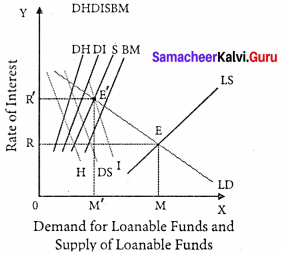Explanation :
X-axis represents the demand for and supply of loanable funds, Y axis represents the rate of interest. The LS curve represents the total supply curve ofloanable funds. The LD curve represents the total demand for loanable funds. The LD and LS curves, intersect each other at the point “E” the equilibrium point. At this point OR rate of interest and OM is the amount ofloanable funds.

Criticisms :

1. Many factors have been included in this theory’. Still there are many more factors like
• Asymmetric information
• Moral Hazard.
2. It is very difficult to combine real factors with monetary factors.

Question 38.
Explain the Keynesian Theory of Interest.
Answer:
According to Keynes, interest is the reward for parting with liquidity’ for a specified period of time.
Meaning of liquidity preference:
Liquidity preference means the preference of the people to hold wealth in the form of liquid cash rather than in other non-liquid assets.
Motives of demand for money:
The three motives of liquidity preference are.

1. The transaction motive Mt = f (y)
2. The precautionary motive Mp = f (y)
3. The speculative motive Ms = f (i)

Determination of Rate of Interest:
According to Keynes, the rate of interest is determined by the demand for and the supply of money. The demand for money is liquidity preference. The supply of money is determined by the policies of the government and the central bank.

Equilibrium between demand and supply of money:
The equilibrium between liquidity preference and demand for money determine the rate of interest. In short-run, the supply of money is assumed to be constant.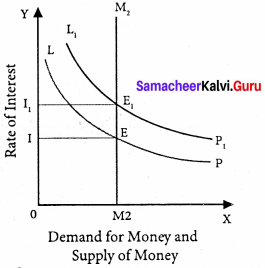LP is the liquidity preference curve. M2 shows the supply curve of money to satisfy speculative motive. Both curves intersect at the point E which is the equilibrium. Here, rate of interest is I.

If liquidity preference increases from LP to L1 P1 the supply of money remains constant, the rate of interest increase from OI to OI1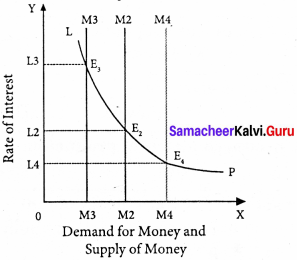Suppose LP remains constant. If the supply of money is OM2, the interest is OI2 and if the supply of money is reduced from OM2 to OM2, the interest would increase from OM2 to OM4. If the supply of money is increased from OM2 to OM4 the interest would decrease from OI2 to Ol4

Criticisms :
1. This theory does not explain the existence of different interest rates prevailing in the market at the same time.
2. It explains interest rate only in the short – run.

## Samacheer Kalvi 11th Economics Distribution Analysis Additional Questions and Answers

Part -A
Choose the best options

Question 1.
Marginal Productivity theory is the …………………….. theory of distribution.
(a) Average
(b) Marginal
(c) Liquidity preference theory
(d) General
Answer:
(d) General

Question 2.
“Interest is the price paid for the use of capital in any market” is said by_______
(a) Adam Smith
(b) Torrance
(c) Walker
(d) Marshall
Answer:
(d) Marshall

Question 3.
The standard of living of workers in a country depends upon the ……………………… wages.
(a) Real
(b) Effective
(c) Direct
(d) Elastic
Answer:
(a) Real

Question 4.
The author of agio-theory of interest.
(a) N.W. Senior
(b) Bohm Bawerk
(c) Walker
(d) Marshall
Answer:
(b) Bohm Bawerk

Question 5.
Who propounded the risk bearing theory of profit?
(a) Bohm – Bawerk
(b) F.B Hawley
(c) Marshall
(d) Walker
Answer:
(b) F.B Hawley

Question 6.
Dynamic theory of profit was propounded by_______
(a) Marshall
(b) J.B.Clark
(c) J.M. Keynes
(d) Walker
Answer:
(b) J.B.Clark

Question 7.
What is meant by MPS?
(a) Marginal production supply
(b) Marginal production sale
(c) Marginal production service
(d) Marginal propensity to save
Answer:
(d) Marginal propensity to save

Question 8.
Time preference theory was given by_______
(a) John Ray
(b) Bohm Bawerk
(c) F.B. Hawley
(d) Irving Fisher
Answer:
(d) Irving Fisher

Question 9.
The demand for labour is ………………………
(a) Effective demand
(b) Direct demand
(c) Derived demand
(d) Elastic demand
Answer:
(c) Derived demand

Question 10.
_______ is the author of modern theory of rent
(a) Joan Robinson
(b) Boulding
(c) Both
(d) None
Answer:
(c) Both

Match the following and choose the answer using the codes given below

Question 1.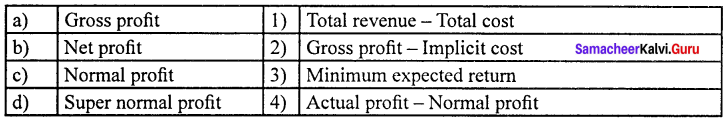(a) 3 4 2 1
(b) 4 3 1 2
(c) 1 2 3 4
(d) 2 3 4 1
Answer:
(c) 1 2 3 4

Question 2.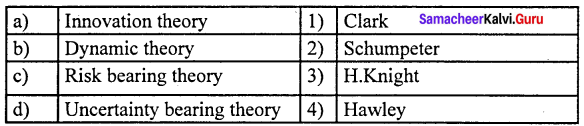(a) 2 1 4 3
(b) 1 4 2 3
(c) 1 2 3 4
(d) 4 1 3 2
Answer:
(a) 2 1 4 3

Choose the incorrect pair

Question 3.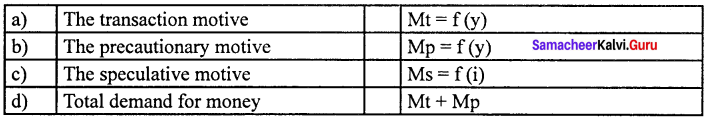Answer:
(d) Total demand for money (iv) Mt + Mp

Question 4.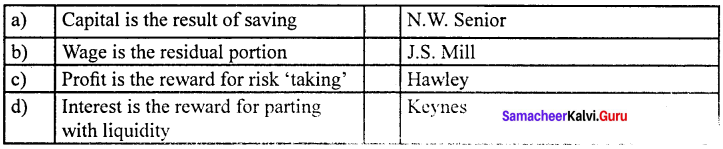Answer:
(b) Wage is the residual portion (ii) J.S. Mill

Choose the correct statement

Question 5.
(a) The supply of land is fixed forever
(b) Quasi-rent accrues to land
(c) The supply of man-made appliances are fixed
(d) Quasi-rent enters price
Answer:
(a) The supply of land is fixed forever

Question 6.
(a) Rent is the reward for labour
(b) Profit is the reward for labour
(c) Wages are the reward for labour
(d) Interest is the reward for organisation
Answer:
(b) Profit is the reward for labour

Choose the odd one out

Question 7.
(a) Bank credit
(b) Hoarding
(c) Dishoarding
(d) Disinvestment
Answer:
(b) Hoarding

Question 8.
(a) Monopoly profit
(b) Wind fall profit
(c) Functional reward
(d) Reward for labourer
Answer:
(d) Reward for labourer

Question 9.
(a) Nominal wages
(b) Real wages
(c) Direct wages
(d) Piece wages
Answer:
(c) Direct wages

Question 10.
(a) Abstinence theory
(b) Loanable funds theory
(c) Liquidity preference theory
(d) Risk bearing theory
Answer:
(b) Loanable funds theory

Analyse the reason for the following

Question 11.
Assertion (A) : Demand for loanable funds depends on investment, consumption and hoarding.
Reason (R) : Supply of loanable funds depends on hoarding and investment.
(a) (A) and (R) are true, (R) is not the correct explanation of (A)
(b) (A) and (R) are true, (R) is the correct explanation of (A)
(c) (A) is true (R) are false.
(d) (A) is false (R) is true.
Answer:
(c) (A) is true (R) are false.

Question 12.
Assertion (A) : The rate of interest is determined by the demand for money and the supply of money.
Reason (R) : The demand for money is liquidity preference.
(a) Both (A) and (R) are true, (R) is not the correct explanation of (A)
(b) Both (A) and (R) are correct, (R) is the correct explanation of (A)
(c) Both (A) and (R) are false.
(d) (A) is false and (R) is true.
Answer:
(a) Both (A) and (R) are true, (R) is not the correct explanation of (A)

Question 13.
Assertion (A) : Only superior land get rent
Reason (R) : Rent arose on account of differences in the fertility of land.
(a) Both (A) and (R) are true
(b) Both (A) and (R) are false correct explanation of (A)
(c) (A) is true and (R) are false.
(d) (A) is false and (R) is true.
Answer:
(a) Both (A) and (R) are true

Choose the incorrect pair

Question 14.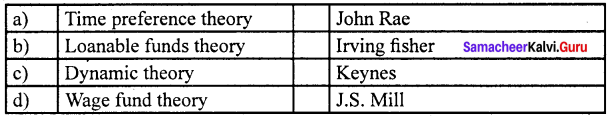Answer:
(d) Wage fund theory (iv) J.S. Mill

Question 15.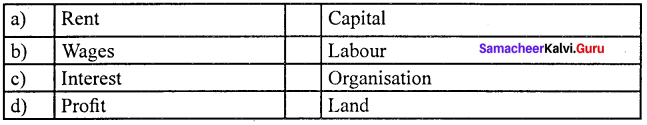Answer:
(b) Wages (ii) Labour

Fill in the blanks with suitable option given below

Question 16.
F.A. Walker wrote a book ______ in 1875.
(a) Political economy
(b) Social economy
(c) Principles of economics
(d) Wealth of nations
Answer:
(a) Political economy

Question 17.
The author of Agio theory of interest______
(a) N.W. Seniors
(b) Bohm-Bawerk
(c) Walker
(d) Marshall
Answer:
(b) Bohm-Bawerk

Question 18.
______ is the produced means of production.
(a) Land
(b) Labour
(c) Capital
(d) Organisation
Answer:
(c) Capital

Choose the best option

Question 19.
The theory of factor prices is popularly known as the theory of
(a) Distribution
(b) Exchange
(c) Wages
(d) Profit
Answer:
(a) Distribution

Question 20.
______ is the author of the modern theory of rent
(a) Joan Robinson
(b) Boulding
(c) Both
(d) None
Answer:
(c) Both

Part – B
Answer the following questions in one or two sentences

Question 1.
What is the theory of distribution?
Answer:
The theory of factor prices is popularly known as the theory of distribution.

Question 2.
Define VMP?
Answer:

1. VMP – means Value of Marginal Product.
2. The Value of Marginal Product is obtained by multiplying the Marginal Physical Product of the factor by the price of product. Symbolically, VMP = MPP x Price.

Question 3.
Define : Marginal productivity theory of distribution.
Answer:
The theory states that price or the reward for any factor of production is equal to the marginal productivity of that factor.

Question 4.
What is marginal product ? Define marginal physical product ?
Answer:
The addition made to the total product by employment of an additional unit of that factor.

Question 5.
Define modern theory of rent.
Answer:
Rent is the difference between the actual earnings of a factor of production and its transfer earning.
Rent = Actual earning – Transfer earning

Question 6.
What is “Wage Fund“.
Answer:
According to Mill, “Every employer will keep a given amount of capital for payment to the workers”. It is known as “Wage Fund”.

Question 7.
Define Quasi Rent.
Answer:
“Quasi rent is the income derived from machines and other appliances made by man
QR = Total revenue – Total variable cost

Part – C
Answer the following questions in one Paragraph

Question 1.
Explain the types of distribution.
Answer:
1. Personal distribution :
It is the distribution of national income among individuals.

2. Functional distribution :
It means the distribution of income among the four factors of production for their services in the production process.

Question 2.
Explain the standard of living theory of wages.
Answer:
This theory of wages was developed by Torrance is an improved version of the subsistence theory of wage. According to this theory, the wage is equal to the standard of living of the workers. If the standard of living is high, wages will be high and vice versa. The standard of a living wage means the amount necessary to maintain the labourer in the standard of life to which he is accustomed.

Question 3.
Briefly explain the Abstinence theory or waiting theory?
Answer:

1. This theory was propounded by N.W.Senior.
2. According to Senior, capital is the result of saving.
3. Saving involves “ abstinence ” or “ Sacrifice
4. It is possible to save only if one abstains from present consumption.
5. Interest is the reward or compensation paid to the saver (capitalist) for his “ abstinence ” or “ sacrifice”.
6. Marshall accepted the Abstinence theory of interest.
7. According to him, interest is a reward for waiting.
8. Saving involves waiting.
9. Therefore, interest is the reward paid to the saver for his “ waiting ”.

Question 4.
State the theories of interest.
Answer:

1. Abstinence theory or waiting theory.
2. Agio theory or the psychological theory.
3. Loanable funds theory or the neoclassical theory.
4. Liquidity preference theory or the monetary theory.

Question 5.
What are the four sources of loanable funds.
Answer:
The supply of loanable funds depends upon the following four sources.

1. Savings (S)
2. Bank Credit (BC)
3. Dishoarding (DH)
4. Disinvestment (DI)

Question 6.
What are the kinds of profit.
Answer:
1. Monopoly profit:
Profit earned by the firm because of its monopoly control.

2. Windfall profit:
Some times, profit arises due to changes in price level. Profit is due to unforeseen factors.

3. Profit as functional reward :
Just like rent, wage and interest, profit is earned by the entrepreneur for his entrepreneurial function.

Question 7.
State the residual claimant theory of wage.
Answer:
This theory was propounded by the American economist F.A. Walkar in 1875, in his book political economy. According to this theory, wage is the residual portion after paying the remuneration of all the other three factors namely land capital and organization.

Criticisms :

1. This theory does not explain the role of trade unions can secure higher wage for workers.
2. Demand side of labour in the determination of wages needs to be considered.

If you need some more information about Tamilnadu State Board Solutions for 11th Economics Chapter 6 Distribution Analysis Questions and Answers then visit our site frequently and get all the resources that you look for. Also, share this page with your friends and make them learn all the concepts thoroughly before the exams.